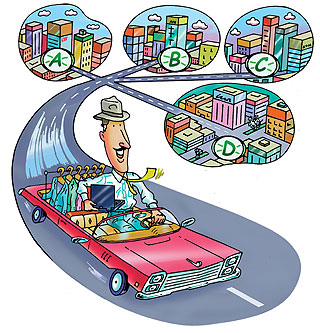# Computational Complexity

 Credits 4 Lecturer Ola Svensson Office hours Wednesdays 14:00 - 16:00 in INJ 112 Schedule Mondays 10-12 in INM 10. Thursdays 8-10 in INR 113.

## Short description

Computational complexity is the part of computer science that studies the computational resources (time, memory, communication, ...) needed to solve problems that we care about. It also looks at the trade-offs and relationships between different types of computation (e.g. whether randomness helps, exact vs approximate solutions, average vs worst case guarantees, ...).

This course familiarizes the students with advanced concepts in computational complexity, and develop an understanding of fundamental questions that underlie some of the key problems of modern computer science. After starting with some basic concepts, potential topics include

• Boolean circuits and nonuniform computation
• Role of randomness in computation
• Interactive proofs and zero-knowledge proofs
• Probabilistically checkable proofs and their characterization of the complexity class NP (PCP Theorem)
• Communication complexity
Recommended book: Sanjeev Arora and Boaz Barak: Computational Complexity: A Modern Approach, Cambridge University Press, 2009. A draft of the book is available for free here.

## Homeworks

• First homework available here. Deadline 17:00 Friday, October 26.
• Second homework available here. Deadline 17:00 Friday, November 16.
• Third homework available here. Deadline 17:00 Friday, December 7.

## Schedule and references

• Lecture 1 (Thursday September 20): Introduction. Diagonalization. Time hierarchy theorem. Chapter 0-3. Another good reference is here. Introductory slides. Ola's notes.
• Lecture 2 (Monday September 24): Nondeterministic computation, P vs NP, and why diagonalization alone won't resolve P vs NP. Chapter 2-3. Recall lecture 2 slides. Ola's notes.
• Exersise session (Thursday September 27) Exercise set 1. Solutions to Exercise set 1.
• Lecture 3 (Monday October 1): Finish proof of why diagnoalization alone won't resolve P vs NP. Beginning of Boolean circuits (non-uniform computation) Chapter 6. Ola's notes.
• Lecture 4 (Thursday October 4): Basic bounds on Boolean circuits and an alternative proof of NP-completeness. Chapter 6. Ola's notes
• Exercise session (Monday October 8) Exercise set 2. Solutions to Exercise set 2.
• Lecture 5 (Thursday October 11): Randomized computation, Adleman's Theorem. Chapter 7. Ola's notes
• Lecture 6 (Monday October 15): Polynomial hierarchy. Karp-Lipton Theorem. Chapters 5 and 6. Ola's notes
• Exercise session (Thursday October 18) Exercise set 3. Solutions to Exercise set 3.
• Lecture 7 (Monday October 22): Natural Proofs. Interactive Proofs. Chapter 8 and 23. Ola's notes
• Lecture 8 (Thursday October 25): Interactive Proofs. Graph Isomorphism. Chapter 8. Ola's notes
• Exercise session (Monday October 29) Exercise set 4. Solutions to Exercise set 4.
• Lecture 9 (Thursday November 1): Interactive Proofs. IP=PSPACE. Chapter 8. Ola's notes
• Lecture 10 (Monday November 5): Final steps of proof of IP=PSPACE and Zero-knowledge proofs Chapter 8. Slides to recall last lecture. Good lecture notes on Zero-knowledge proofs here (Sections 1-2) and here.
• Exercise session (Thursday November 8) Exercise set 5. Solutions to Exercise set 5.
• Lecture 11 (Monday November 12): Probabilistic Checkable Proofs. Local Testability and Decodability of Walsh-Hadamard Code Chapter 11. Ola's notes
• Lecture 12 (Thursday November 15): Probabilistic Checkable Proofs. NP is a subset of PCP(poly(n), 1). Chapter 11. Ola's notes
• Exercise session (Monday November 19) Exercise set 6. Solutions to Exercise set 6.
• Lecture 13 (Thursday November 22): Probabilistic Checkable Proofs. Hardness of approximation. Chapter 11. Ola's notes
• Lecture 14 (Monday November 26): Cryptography: one-way functions and pseudorandom generators. Chapter 9. Ola's notes
• Exercise session (Thursday November 29) Exercise set 7. Solutions to Exercise set 7.
• Lecture 15 (Monday December 3): Cryptography: proof one-way permutations imply PRGs. Chapter 9. Ola's notes
• Lecture 16 (Thursday December 6):Definition of Quantum computing. Chapter 10. Ola's notes
• Lecture 17 (Monday December 10):Continuation of quantum computing: Grover's search algorithm. Chapter 10. Ola's notes
• Exercise session (Thursday December 13) Exercise set 8. Solutions to Exercise set 8.
• Lecture 18 (Monday December 17):Repetition for exam. Final Exam in similar course given 2016. Solutions to that exam.
• Exam (Thursday December 20)